Tutorial¶

This tutorial will walk you through the steps to repeat the analysis of the AGN PG1553+113 done in the official Fermi collaboration python tutorial and results published here.

We strongly recommend that you run this analysis once, so that you can be sure to produce correct results before analyzing your source.

Make a new directory now where you want all of your intermediate and final analysis results to be placed and go there. For illustration purposes we will assume in this tutorial this directory is ~/myanalysis:

mkdir ~/myanalysis`~/myanalysis`
cd ~/myanalysis

First get the data as explain the the offical page. You should have this when typing ls

L110728114426E0D2F37E85_PH00.fits
L110728114426E0D2F37E85_PH01.fits
L110728114426E0D2F37E85_SC00.fits

Make an ascii will with the ‘entire’ path of the FT1 (PH) fits files. Let’s assume this file is called data.list .

The analysis can be performed on disjoint time bins. An ascii file must be provided in the [Time]/file option. The file must be a 2 columns file with T_start and T_stop (in MJD, MET or JD). Each line is a time bin.

239557418 239657418
239667418 239757410
239957418 239967418

Folder management¶

Once you have decided where the results will be placed (here ~/myanalysis), enrico will create some subfolders to store results and files.

• fits files (livetime cube, selected events, etc…) will be placed in the main folder (here ~/myanalysis)
• The spectrum results (ascii files, plots) will be stored in a folder named Spectrum (here ~/myanalysis/Spectrum)
• Data (fits files) and results from the generation of energy bin will be stored in a subfolder Ebin# where # is the number of bins you ask for (here ~/myanalysis/Ebin#).
• Data (fits files) and results generated for the light curve will be placed in Lightcurve# where # is the number of bins you ask for (here ~/myanalysis/Lightcurve#).
• TSmap data will be placed in a TSmap subfolder (here ~/myanalysis/TSmap).

Make a config file¶

enrico uses configuration file to run analysis. The way it has been thought, you need only one configuration file per analysis. The drawback is that such file can be complicated to generated by hand or to understand (for a description see Configuration Files).

You can use the enrico_config tool to quickly make a config file called myanalysis.conf. It will ask you for the required options and copy the rest from a default config file enrico/data/config/default.conf:

\$ enrico_config myanalysis.conf
Please provide the following required options [default] :
Output directory [~/myanalysis] :
Target Name : PG155+113
Right Ascension: 238.92935
Declination: 11.190102
redshift, no effect if null  : 0.49
ebl model to used
0=Kneiske, 1=Primack05, 2=Kneiske_HighUV, 3=Stecker05, 4=Franceschini, 5=Finke, 6=Gilmore : 4
Options are : PowerLaw, PowerLaw2, LogParabola, PLExpCutoff
Spectral Model [PowerLaw] : PowerLaw2
ROI Size  : 10
FT2 file [~/myanalysis/spacecraft.fits] : ~/myanalysis/L110728114426E0D2F37E85_SC00.fits
FT1 list of files [~/myanalysis/events.lis] : ~/myanalysis/data.list
tag [LAT_Analysis] : TestOfPG1553
Start time  : 239557417
End time  : 256970880
Emin  : 200
Emax  :
IRFs [CALDB] :
evclass  :
evtype  :
Corresponding IRFs   =       ('P8R2_SOURCE_V6', ['BACK', 'FRONT'])
Is this ok? [y] : y
Corresponding zmax =  95

Note :

• Always give the full path for the files
• We used the PowerLaw2 model as in the Fermi tutorial.
• Time is give in MET
• Energy is given in MeV
• ROI size is given in degrees

Now you can edit this config file by hand to make further adjustments. For more informations about the configuration file see Configuration Files

Note

If you know exactly how the analysis steps work you can also make adjustments later on. But we have not put in a gadzillion of checks for each step to make sure that parameters are consistent with previous steps, so it is best to only adjust parameters at the beginning.

Make a model xml file¶

The ST works using an sky model written in xml format. Often, this model is complicated to generate. You can run enrico_xml to make such model of the sky and store it into a xml file which will be used for the analysis.

\$ enrico_xml myanalysis.conf
use the default location of the catalog
use the default catalog
Use the catalog :  /CATALOG_PATH/gll_psc_v06.fit
Add  12  sources in the ROI of  10.0  degrees
3  sources have free parameters inside  3.0  degrees
Iso model file  /gpfs/LAPP-DATA/hess/sanchez/Fermi/Soft/ScienceTools//IExternal/diffuseModels/v5r0/iso_P8R2_SOURCE_V6_v06.txt
Galactic model file  /gpfs/LAPP-DATA/hess/sanchez/Fermi/Soft/ScienceTools//IExternal/diffuseModels/v5r0/gll_iem_v06.fits

write the Xml file in  ~/myanalysis/PG155+113_PowerLaw2_model.xml

Note

Note that you give options for this step simply by mentioning the config file. For the enrico_xml tool, the relevant options are in the [space], [target] section. The out file is given by [file]/xml.

The Iso model can be guess using the evclass and evtype given in the config file. If the file does not excist the default one is used

Get data¶

There are two possibilities:

• Use the weekly generated fits file available on the web

Run global fit¶

The gtlike tool implemented in the ST find the best-fit parameters by minimizing a likelihood function. Before running gtlike, the user must generate some intermediary files by using different tools. With enrico, all those steps are merged in one tool. To run the global fit just call :

\$ enrico_sed myanalysis.conf

This will make all the steps for you (gtselect, gtmktime,gtltcube, etc…), produce all the required fits files and fit the data (gtlike). A file with the extension ‘results’ will be produced and where all the results will be stored.

If you want to refit the data because e.g. you changed the xml model, you are not force to regenerate the fits file. Only the gtlike tool should be recall. This is also possible with enrico. By changing the option [spectrum]/FitsGeneration from yes to no, enrico will be told to not generate the fits files and directly proceed to the fit.

if the found TS is below the value set in [UpperLimit]/TSlimit, then an upper limit is computed.

Note

For the enrico_sed tool, most of the relevant options in the [spectrum] section

You can use enrico_testmodel to compute the log(likelihood) of the models POWERLAW, LogParabola and PLExpCutoff. An ascii file is then produced in the Spectrum folder with the value of the log(likelihood) for each model. You can then use the Wilk’s theorem to decide which model best describe the data.

Make flux points¶

Often, an SED is presented with point obtained by restricting the energy range and re-run a complete analysis.

To make flux points, again enrico_sed tool will be used. It will first run a global fit (see previous section) and if the option [Ebin]/NumEnergyBins is greater than 0, then at the end of the overall fit, enrico will run NumEnergyBins analysis by dividing the energy range.

Each analysis is the a proper analysis (it runs gtselect, gtmktime,gtltcube,…, gtlike), run by the same enrico tool than the full energy range analysis. If the TS found in the time bins is below [Ebin]/TSEnergyBins then an upper limits is computed.

Note

If a bin failed for some reason or the results are not good, you can rerun the analysis of the bin by calling enrico_sed and the config file of the bin (named SOURCE_NumBin.conf and in the subfolder Ebin#).

Note

Most of the relevant options in the [Ebin] section.

Make a light curve¶

A light curve is obtained by run the entire analysis chain into time bins. To make a light curve

\$ enrico_lc myanalysis.conf

It will divide the time range in [LightCurve]/NLCbin bins and run a proper analysis. If the TS found in the time bins is below [LightCurve]/TSLightCurve then an upper limits is computed.

Note

Note that you give options for this step simply by mentioning the config file. For the enrico_lc tool, most of the relevant options are in the [LightCurve] section

It is also possible to make a folded LC using

\$ enrico_foldedlc myanalysis.conf

This is designed for binary or periodic system. The user provide the starting point a period and the period lentgh as well as number of LC bins

Make a TS map¶

TS map are use to find new source in a ROI. They are produced by adding a spurious source on each point of a grid (pixel) and computing the TS of this source.

You can make a TS map using the tool enrico_tsmap. It will compute the TS in each bin of the ROI. You must have run enrico_sed before.

Note

This binning is based on the count map produced during the fit of the full energy range enrico_sed. The division of the ROI controlled by the option [space]/npix and [space]/npiy but cannot be change after having run enrico_sed.

In order to speed up the process, parallel computation can be used. Either each pixel can be a job by itself (option [TSMap]/method = pixel) or a job can regroup an entire row of pixel (option [TSMap]/method = row)

If you want of remove the source your are interested in from the TS map (i.e. froze its parameters to the best fit values) use [TSMap]/RemoveTarget = yes.

Note

For the enrico_tsmap tool, most of the relevant options are in the [TSMap] section

It can happend that some job failed of the obtain TS is not good (due to convergence problems) If a pixel (or a row) has failed, you can rerun it.

To re-run a single pixel, i.e. the pixel (49,4) :

enrico_tsmap myanalysis.conf 49 4

To re-run a row, ie row number 49 :

enrico_tsmap myanalysis.conf 49

Upper Limits¶

An upper limits is calculated if the Test Statistic (TS) of the source is below a certain limit set by the user. To set this limit for :

• enrico_sed, use the option [UpperLimits]/TSlimit.
• bins in energy, use the option [Ebin]/TSEnergyBins.
• enrico_lc, use the option [LightCurve]/TSLightCurve.

3 methods are available :

• The profile method, which look for a decrease of a certain amount of the likelihood function
• The integral method which compute the integral of the likelihood function as a function of a parameter to set the UL
• The Poisson method based on Feldman-Cousins method for low signal

The first 2 implementations are provided by the ScienceTools and used by enrico.

Note

For upper limits, most of the relevant options are in the [UpperLimits] section

Results¶

At the end of enrico_sed, a file with the extension .results is created. After is an example for a POWERLAW model:

• Index : Value of the spectral index (for POWERLAW)
• Scale : Value of the scale parameter (MeV, for POWERLAW)
• Optimizer : optimizer used in the fit
• Emin : Value of the energy min (MeV)
• Emax : Value of the energy max (MeV)
• tmax : Value of the time max (MET)
• dScale : Error of the scale parameter (MeV, for POWERLAW)
• TS : Value of the Test statistic
• ModelType : Spectral model fitted
• SrcName : Name of the target
• Flux : fitted integral flux (ph/cm2/s)
• Npred : Number of predicted photons
• log_like : Value of the loglikehihood
• dIndex : Error of the spectral index (for POWERLAW)
• dFlux : Error fitted integral flux (ph/cm2/s)
• dPrefactor : Error Prefactor parameter (ph/MeV/cm2/s)
• tmin : Value of the time min (MET)
• Prefactor : Value Prefactor parameter (ph/MeV/cm2/s)

Summaries of the LC and Ebin computations are also given in a ascii file at the end of enrico_plot_sed and enrico_plot_lc

Plot results¶

Now, we want to plot the results of the analysis we performed. Some plots can be produced by enrico. Using the tools enrico_plot_* allow to plot the results of your analysis.

The 1 sigma contour plot can be computed by enrico_sed if the option [Spectrum]/ResultPlots=yes. Then to plot it, call enrico_plot_sed myanalysis.conf which will make a SED with the 1 sigma contour and add the data points computed previously (section Make flux points).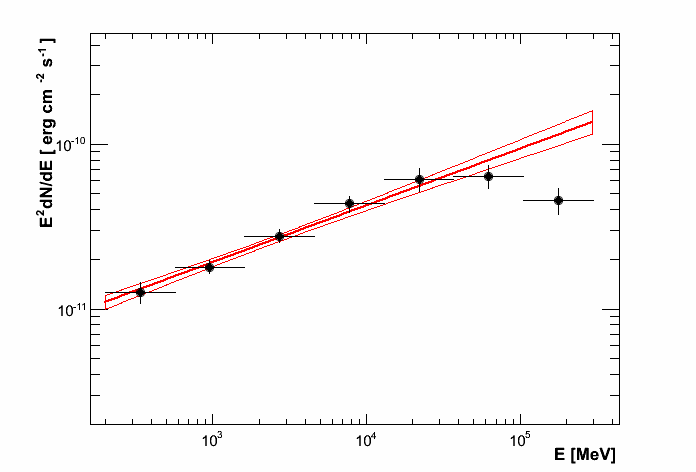SED of PG 1553+113

If you ran a binned analysis and with the option [Spectrum]/ResultPlots=yes then a model map is produced to compare with the real count map (see the section check results).

• The light curve can be plotted using enrico_plot_lc myanalysis.conf as well as diagnostic plot (TS vs time, Npred vs time, etc…)
Chi2 =  33.4499766302  NDF =  19
probability of being cst =  0.0213192240717

Fvar =  0.17999761777  +/-  0.089820202452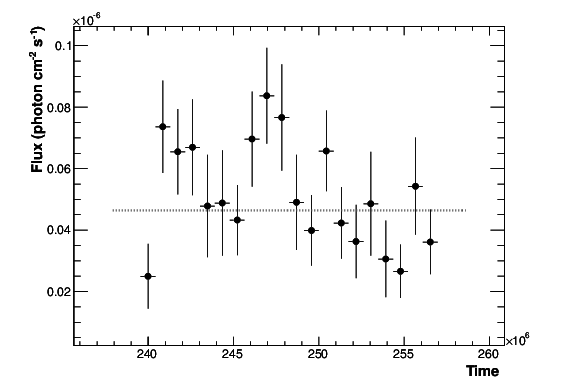Light curve of PG 1553+113. The dashed gray line is the results of a fit with a constant.

enrico also computes the variability index as described in the 2FGL catalog (see here).

• The TS map (see the section Make a TS map) can be plotted and save in a fits file using enrico_plot_tsmap myanalysis.conf. This will generate a fits file that can be plotted using the script plotTSmap.py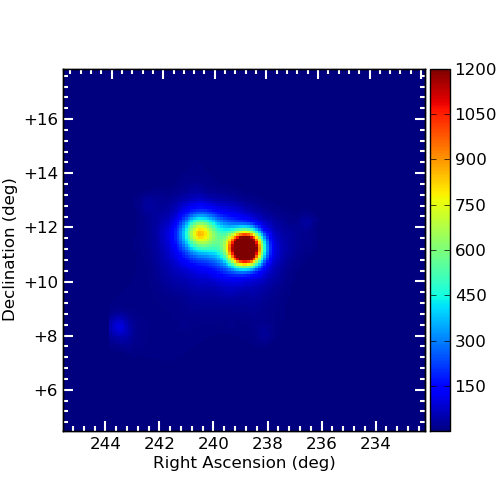TS Map of PG 1553+113.

Check results¶

There is different way to check the quality of a results. First have a look the log file and loff for any error or warning messages. Enrico also produce maps that can use to check the results

Spectrum

• counts map, model map and subtract map.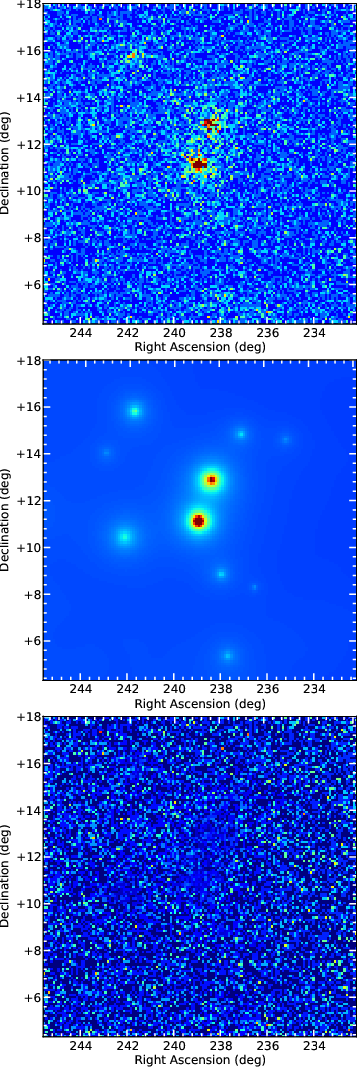Maps of PG 1553+113, from top to bottom: counts map, model map, residuals map.

These maps are use to visualize the ROI and check and see any misfitted sources. You can plot then using the script ‘plotMaps.py’

• Counts Plot and Residuals. The points (# counts/bin) are the data, and the solide line is the source model. Dashed line is the sum of all other model and dotted line is the sum of both. Error bars on the points represent sqrt(Nobs) in that band, where Nobs is the observed number of counts. The Residuals are computed between the sum model and the data.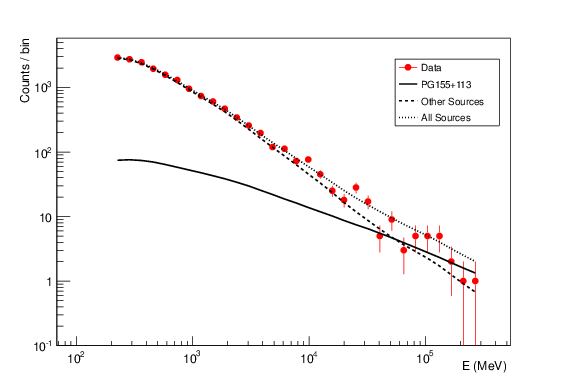Count plot of PG 1553+113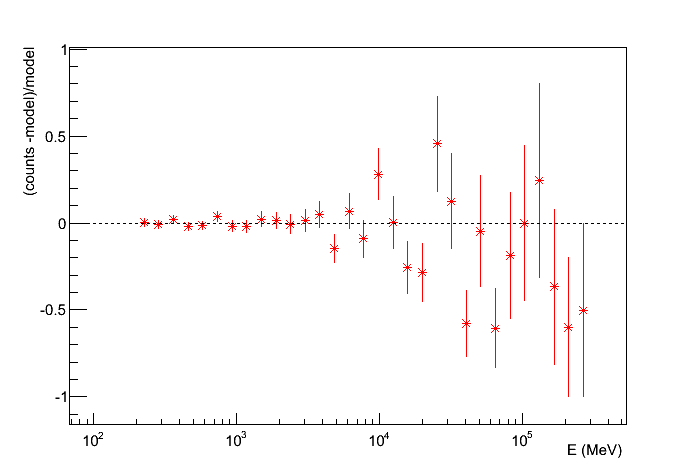Residuals plot of PG 1553+113

Light-curves

• The generation of light-curves might suffer from troubles, especially in the error bars computation. To check this, enrico plots the flux/dflux vs Npred/DNpred. If the errors are well computed the two variables are highly correlated.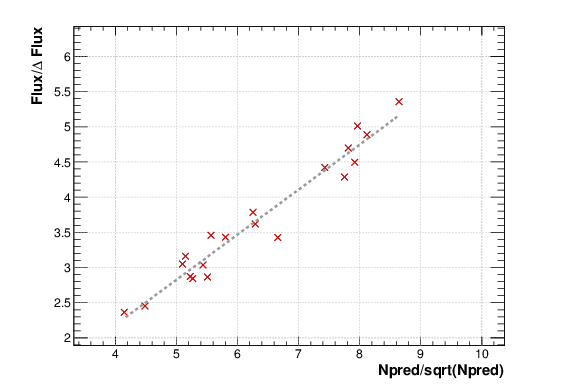flux/dflux vs Npred/DNpred plot of PG 1553+113. Red points are time-bins with TS> TSlimit, black (if any) are point for which an upper-limits on the flux was calculated and the points can be safely ignored in this plot. The gray dashed line is the fit with a linear function. to guide the eyes.

Make Profile likelihood¶

The tool enrico_scan allows to make a profile likelihood of the free parameters of the target. Each plot is save under the scan folder. Fits files must have been generated before.

Make Confidence contour¶

The tool enrico_contour allows to make a contour of 2 free parameters of the target. Each plot is save under the contour folder. Fits files must have been generated before.

Get the highest energy events¶

The tool enrico_srcprob compute the highest energy photons that can be associated to a list of sources (provided in an ascii file). The probability of the photons to be associated to the sources is also computed

Refine source position¶

The tool enrico_findsrc is based on gtfindsource and find the best position of a source

Check different models¶

enrico_testmodel will run the gtlike analysis with different model and compute the loglikehihood value for each model. The user can then decide, base on a LRT which model best describe the data. Fits files must have been generated before.

Test a particular model list¶

enrico_lrt will run the gtlike analysis with a (list of) model(s) given as lines in a models file. The user can decide which parameters are to be fixed (by specifying a value) and which ones are going to be free (None value).

Lines should contain something like: LogParabola, None, 1.571613825, 0.06406550853

Then we call the lrt macro by running:

enrico_lrt mysourceconfig.conf mylistofmodels.txt

If no list of models is specified, enrico_lrt will execute the routines in enrico_testmodel.

Results and files will be saved to the /TestModel/ subdirectory. The .results file contains the likelihood value appended to the end of the model. Please note that the formatting is slightly different than in enrico_testmodel (comma separated instead of tab separated values)